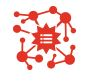010-68421378
sales@cogitosoft.com

# MATHEMATICA

WOLFRAM MATHEMATICAMathematica 在其 30 年的开发历程中，在技术计算领域确立了最先进的技术，并为全球技术创新人员、教育工作者、学生和其他人士提供了最主要的计算环境。

Mathematica 以卓越的技术和简便的使用方法享誉全球，在此基础上，它提供了单个集成并且持续扩展的系统，涵盖了最广最深的技术计算功能。现在利用 Mathematica Online（在线），它还能通过网页浏览器实现云端的完美访问，以及在所有现代桌面系统上的本地访问。Mathematica 具有涵盖所有技术计算领域的将近 5,000 个内置函数——所有这些都经过精心制作，使其完美地整合在 Mathematica 系统中。

Mathematica 在所有领域构建了前所未有的强大算法—许多算法都是使用 Wolfram 语言独特的开发方法和功能进行构建的Mathematica 使用 Wolfram 笔记本界面，使您可以快速整理包括文本、可运行代码、动态图形和用户界面等的丰富文档中的任何内容。

Mathematica 使用最先进的计算美学和设计原理，为你呈现最美观的结果；立即创建最顶级的互动可视化效果和出版物质量级别的文档。Mathematica 目前已经完美地集成于云端系统中；可在统一强大的云端桌面混合环境中进行分享、云计算以及更多功能。Mathematica 凭借它的算法功能以及 Wolfram 语言的详细设计原理，创建了具有预测性建议、自然语言输入等的独特的并且易于使用的系统。Mathematica 可以访问广博的 Wolfram 知识库，包括最实时的数千个领域的数据。Mathematica 为与任意内容连接而构建：文件格式（180 多种）、其他语言、 Wolfram Data Drop、API、数据库、程序、物联网和设备，甚至其自身分布等

Mathematica 基于具有突破性意义的 Wolfram 语言。Wolfram 语言Wolfram 笔记本界面Wolfram CloudWolfram 算法库Wolfram EngineWolfram KnowledgebaseMathematica 发展轨迹1988 年革新

Mathematica 在 30 年多的时间里遵从快速革新的发展轨迹，使得在每个阶段都构建了许多强大的新功能。

Mathematica 的各版本更新不仅仅是一般的软件更新；每个连续更新的版本都是在新方向上对计算模式的一次重大发展，并且引入了重要的新思路。

30 年多来的持续发展

Mathematica 忠实于它的核心准则和严肃的设计原理，持续发展并且集成了许多新功能和方法，而无需走回头路。

Mathematica 是 Wolfram 独创的旗帜产品；旨在为研发和教育工作提供技术计算平台。基于 Wolfram 语言，Mathematica 与其他核心 Wolfram 产品可以百分百兼容。京公网安备 11010802021106号网络文化经营许可证京网文4225-497号 | 违法和不良信息举报电话：4006561155

© Copyright 2000-2020 北京哲想软件有限公司版权所有 | 地址:北京市海淀区西三环北路50号豪柏大厦C2座11层1105室

北京哲想软件集团旗下网站：哲想软件 | 哲想动画